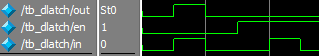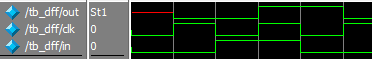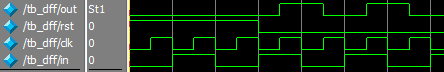版权申明：本文为博主窗户(Colin Cai)原创，欢迎转帖。如要转贴，必须注明原文网址

http://www.cnblogs.com/Colin-Cai/p/12045295.html

作者：窗户

QQ/微信：6679072

E-mail：6679072@qq.com

上一章给出了组合电路的仿真实现，这一章开始思考时序电路的仿真实现。但时序电路远比组合电路复杂的多，我们先从组成电路的每个元件说起。在程序实现层次，我们可以考虑给每个基础元件一个自定义描述方式，称为原语。

Verilog原语

Verilog提供了元件原语建模的方式，说白了，就是用一个表格来体现所有情况下的输出。Verilog的原语只允许有一个输出。

比如and门，用Verilog原语来描述如下

primitive myand(out,in1,in2);
output out;
input in1,in2;
table
// in1 in2    out
0   ?   :  0;
1   0   :  0;
1   1   :  1;
endtable
endprimitive

Verilog原语中不能使用高阻(因为除了三态门产生高阻输出之外，这的确与真实电路不符，而Verilog并无VHDL那般抽象)，不能表示三态门。

对于时序电路，Verilog也一样可以支持。所谓时序电路，意味着电路的输出不仅仅与当前电路的输入有关，还与电路之前的状态有关，所谓电路之前的状态也就是电路之前的输出。

我们来考虑这样一个时序元件，称之为D锁存器，有两个输入en和in，一个输出out。当en为0时，out和in保持一致；当en为1时，out保持不变。这称之为电平触发。用波形图可以描述其特性：用verilog描述可以如下：

module dlatch(out, en, in);
output out;
input en, in;
reg out;
always@(in)
if(!en)
out <= in;
endmodule

电平触发的D锁存器可以用原语描述如下：

primitive dlatch(out, en, in);
output out;
input en, in;
reg out;
table
//en in : out : next out
0   0 :  ?   :  0;
0   1 :  ?   :  1;
1   ? :  ?   :  -;
endtable
endprimitive

状态表的最后一行next out位置的 - 符号代表状态保持。

再来一个我们数字设计时最常用的元件D触发器，它有两个输入信号clk和in，有一个输出信号out。当clk从0变到1的瞬间(称之为上升沿)，out被赋予in的值，其他时候out保持不变。这种触发称之为沿触发。波形图可以用以下描述其特性：用Verilog描述如下：

module dff(out, clk, in);
output out;
input clk, in;
reg out;
always@(posedge clk)
out <= in;
endmodule

而用Verilog原语描述则如下：

primitive dff(out, clk, in);
output out;
input clk, in;
reg out;
table
// clk  in : out : next out
(01) 0  :  ?   : 0;
(01) 1  :  ?   : 1;
endtable
endprimitive

原语没有写的部分都是保持。换句话说，之前D锁存器的原语实现table的最后一行保持是可以不写的。

前面的D锁存器是电平触发，D触发器是沿触发。实际上原语也可以同时支持两种触发。比如存在异步复位的D触发器，多了个触发的rst信号，在rst为1的时候，out会被赋予0。波形图如下：Verilog描述可以如下：

module dff(out, rst, clk, in);
output out;
input rst, clk, in;
reg out;
always@(posedge rst or posedge clk)
if(rst)
out <= 1'b0;
else
out <= in;
endmodule

用原语描述则为：

primitive dff(out, rst, clk, in);
output out;
input rst, clk, in;
reg out;
table
// rst clk  in : out : next out
0 (01) 0  :  ?   : 0;
0 (01) 1  :  ?   : 1;
1  ?   ?  :  ?   : 0;
endtable
endprimitive

以上的原语中就同时包含电平触发和沿触发。

Scheme建模下的原语

Verilog原语用表来表示，实际上是用表来代表一个函数关系，于是我们要做的，是试着用一个函数来代表基本元件的原语描述。

比如与门，我们是不是可以用以下函数来描述:

(define (myand in1 in2)
(if (and (= in1 1) (= in2 1)) 1 0))

上述函数方便的表示一个组合逻辑，甚至上述可以延伸到表示任意多输入的一个与门，描述如下

(define (myand . in)
(if (member 0 in) 0 1))

可是上述的描述并未方便的引入时序的概念，最终在仿真的时候无法区分组合逻辑和时序逻辑。从而上述的函数来代表原语描述是失败的，需要再修改一下。

于是我们描述函数的参数列表里不仅有当前各输入信号，还得有当前输出信号，考虑到沿触发器件，还得加入沿的信息。于是我们可以定义原语是这样的一个函数：带有三个参数，第一个参数是输入信号值的列表，第二个参数是当前输出信号值，第三个参数代表沿触发的信号，简单起见，就用沿触发的信号在输入信号列表中的序号来表示，如果不是沿触发则此处传入-1；函数返回即将输出的信号值。

那么我们的任意多输入的与门，描述如下

(define (myand input current-output edge-)
(if (member 0 input) 0 1))

那么D锁存器的原语描述如下

(define (dlatch input current-output edge)
(let ((en (car input)) (in (cadr input)))
(if (= en 1) current-output
in)))

上面的let显示了输入列表是[en, in];

D触发器的原语描述如下，输入列表为[clk, in]

(define (dff
(let ((clk (car input)) (in (cadr input)))
(if (and (= edge 0) (= clk 1)) in
current-output)))

对于之前带异步复位的D触发器，作为一个既有电平触发又有沿触发的例子

(define (dff-with-rst input current-output edge)
(cond
((= rst 1) 0)
((and (= edge 0) (= clk 1)) in)
(else current-output))))

进一步修改原语

之前的设计已经完备，但未必方便。比如可能一些逻辑可编程器件的编程粒度不会细到门级。Verilog的原语里，只有一个输出，我们可以考虑这里原语的输出可以有多个。

在此我们考虑一位全加器，也就是三个单bit的数相加，得到两位输出的组合电路，输出信号既然可能不止一个，原语函数的输出当然是一个列表，第二个参数current-output当然也是列表。

(define (add input current-output edge)
(let* ((sum (+ a b c)) (cout (if (>= sum 2) 1 0)) (s (if (= cout 0) sum (- sum 2))))
(list cout s))))

最后，我们考虑，原语可以为每一个信号可以加一个位宽。

在这里，我们来考虑做一个四位计数器，有一个异步复位(rst)，有一个时钟(clk)，一个4位的输出(out)，每当clk上升沿，输出都会加1，注意如果当前输出如果是1111，下一个输出将会是0000，描述如下

(define (counter input current-output edge)
(cond
((null? lst) '())
((= (car lst) 0) (cons 1 (cdr lst)))
(else (cons 0 (add1-list (cdr lst))))))
(let ((rst (car input)) (clk (cadr input)))
(cond
((= rst 1) '((0 0 0 0)))
((and (= edge 1) (= clk 1)) (list (add1-list (car current-output))))
(else current-output))))

用0/1的list有一些不方便的地方，我们可以用数来代替，也可以考虑数和list一起支持，那么我们在处理的时候可能需要判断一下传入的是数还是list，Scheme里提供了两个函数来判断，一个是list?用来判断是不是list，一个是number?用来判断是不是数。在上面定义的基础上加上对于数的支持也很容易。

迭代

以上虽然用函数来定义了原语，但是从函数却没有定义任何表示原语信号接口的东西，不看原语定义无法知道原语怎么使用，并且在仿真的时候，上述原语本身并不提供各个信号当前的值。

本来会在后面的章节提到解决方案，在此也给个方案。

我们可以用闭包解决这个问题，闭包中包含着输入、输出信号的信息。Scheme的闭包可以有多种方式，可以采用上一章中局部作用域变量的方法(这种方法并不是所有的语言都支持，比如Python则只能用class建立类了)，另一种方式则是用不变量了，也就是纯函数式编程方式。本章就来说说第二种方式，虽然在我之前的其他文章中说到的闭包主要是采取这种方式。

我们先看一个简单的例子，我们希望有这样的需求：

定义一个变量x

(define x (make-sum 0))

(set! x (x 1))

(set! x (x 2))

(set! x (x 3))

(x)得到6

这样，每次x都是一个闭包，现在要看如何定义make-sum。

我们先这样定义:

(define (make-sum n)
(lambda (m)
(make-sum (+ n m))))

但是，我们马上发现，我们要求的值变的不可提取，闭包返回的这个函数，不仅仅可以带一个参数用来再度返回闭包，还应该可以不带参数，以支持上面(x)这样的提取。

上面的实现需要一点修改，需要判断一下参数个数：

(define (make-sum n)
(lambda s
(if (null? s) n
(make-sum (+ n (car s))))))

测试一下，OK了，最后得到了6,说明make-sum是可行的。

然后，我们可以抽象加法这个符号，继续做算子f-step。

(define (f-step step n)
(lambda s
(if (null? s) n
(f-step step (step n (car s))))))

这样，make-sum可以由上述算子定义而得

(define make-sum
(lambda (n) (f-step + n))

定义f-step算子有什么好处呢？实际上，它是为迭代的每一步动作进行建模。

于是我们可以用f-step为零件，构建所有的迭代。

比如对于辗转相除法(欧几里得算法)求最大公约数，描述如下

(define (gcd a b)
(if (zero? b) a
(gcd b (remainder a b))))

如果要用f-step，则首先要把迭代的内容表示成一个对象，可以用cons对来对gcd的两个参数a,b打包。

f-step的第二个参数是一个函数，我们称之为step，step函数有两个参数，一个是用于迭代的数据，在这里就是这个cons对，而第二个参数可以看成是外界激励，这里是不需要的，传任意值即可。

我们清楚辗转相除法的这一步，应该描述如下

(define (step pair none)
(cons (cdr pair) (remainder (car pair) (cdr pair))))

反复的迭代，其终止条件是判断pair的第二个成员是否为0,如果是0则返回pair的第一个成员，否则继续迭代

(define (continue-gcd-do f)
(let ((x (f)))
(if (zero? (cdr x)) (car x)
(continue-gcd-do (f '())))))

于是，我们的gcd就被重新设计了

(define (gcd a b)
(continue-gcd-do (f-step step (cons a b))))

虽然看起来的确比最开始的实现复杂了不少，但是可以实现统一的设计，以便更复杂的情况下的应用。

反柯里化

f-step还可以用来设计fold-left算子，我们回忆一下fold-left

(fold-left cons 'a '(b c d))

得到

'(((a . b) . c) . d)

我们可以看成是一个迭代，

最开始是'a

然后通过函数cons和'b，得到

'(a . b)

然后再通过函数cons和'c，得到

'((a . b) . c)

最后再通过函数cons和'd，得到

'(((a . b) . c) . d)

显然，我们可以使用f-step，定义以下

(define func (f-step cons 'a))

那么

(((func 'b) 'c) 'd)

则是最后的结果。

但这样似乎不太好用，假如我们有这么一个函数，暂且称为F

((F func) 'b 'c 'd)

也就是

(apply (F func) '(b c d))

那么就容易实现了。

F这个过程正好和我之前的文章《map的实现和柯里化(Curring)》里的柯里化过程相反，称之为反柯里化，重新给个合适的名字叫uncurry

(define (uncurry f)
(lambda s
(if (null? s) (f)
(apply (uncurry (f (car s))) (cdr s)))))

于是fold-left就可以如下实现

(define (my-fold-left f init lst)
(apply (uncurry (f-step f init)) lst))

封装

绕了一圈，似乎与主题有点远了。一个原语所表示的电路，实际上也是随着外界输入，在不断的变化输出，也可以用f-step算子来模拟。

电路的状态包含了电路的输出，同时也包含着电路的输入，因为需要判断沿变化，当然我们只需要关注沿触发的信号就行了，其他输入信号不需要在状态里。

我们就以之前的带复位的D触发器为例，我们重新给出它的原语描述，并按第三节里修改之后的来，

(define (dff-with-rst input current-output edge)
(cond
((= rst 1) '((0)))
((and (= edge 0) (= clk 1)) (list (list in)))
(else current-output))))

我们的初始状态可以设置为

'((z) . (z))

之所以用z来表示，而不是0/1，在于初始的时候，我们认为都是一种浑沌的状态，当然，也可以设为用0/1，这完全可以按仿真意愿来。

前面第一个'(z)表示所有可以带来沿触发的信号列表，这里可以带来沿触发的是第二个信号clk，序号从0开始算为1，而输出信号初始也先设置为'(z)

于是状态转换函数则为

(define step (lambda (stat input)  (cons (cadr input)
(dff-with-rst input (cdr stat)
(if (eq? (caar stat) (caadr input)) -1 1)))))

于是

(f-step step '(() . ()))则是一个原语的实例封装，里面包含着状态，可以用来在仿真中反复迭代。

posted on 2020-01-20 11:21  窗户  阅读(759)  评论(0编辑  收藏  举报